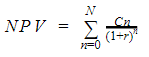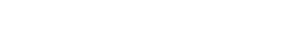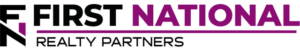# Net Present Value (NPV) vs. Internal Rate of Return (IRR)

### Key Takeaways

• Two commonly used metrics to evaluate a commercial real estate investment are the Net Present Value (NPV) and Internal Rate of Return (IRR).
• The NPV is the summation of the property’s cash flows discounted back to the present using a required rate of return.
• The IRR is the rate of return on each dollar invested, for the time period it is invested in.

Two commonly used commercial real estate metrics are the Net Present Value (NPV) and Internal Rate of Return (IRR).  The calculation of these metrics has some overlap so it is critically important to understand the difference between them and how they contribute to the ultimate investment decision.

## What is the Net Present Value?

NPV is defined as the net present value of a series of cash flows, discounted by the investor’s required rate of return.  By looking at the money an investor expects to earn from a property and translating those funds into a present dollar amount, a decision can be made as to whether or not the investment is worthwhile.

The formula used to calculate NPV is:In the formula, “C” represents the cash flow earned in each period, “n” represents each period, “N” is the total holding period, and “r” is the investor’s chosen discount rate.

If the result of the NPV calculation is a number other than zero, it indicates that the property is failing to achieve the chosen discount rate and the exact value indicates how far off it is from achieving the target yield.  This can be seen in the example below.

## What is the Internal Rate of Return?

The internal rate of return is the rate of interest earned on each dollar invested for each period of time that it is invested in.  Mathematically, it is also the discount rate that sets the net present value of all future cash flows equal to zero.  In other words, if the discount rate chosen in the NPV calculation and the IRR are the same, the NPV is equal to zero.

## NPV vs IRR – Key Differences

Although the two metrics provide similar information, there are three key differences:

1. NPV attempts to calculate the present value using chosen discount rates or “cut off rates.”  The IRR calculation discounts cash flow using a trial and error calculation to determine the rate of return.
2. The chosen discount rate using the NPV method takes into account an investor’s cost of capital.  This is not the case with IRR.
3. Finally, the NPV calculation assumes that cash flows are reinvested at the discount rate while the IRR method assumes that cash flows are discounted at the prevailing internal rate of return.

When comparing two potential investments to each other, it is important to be aware of these differences and to take them into account when considering whether or not to proceed with a purchase.

## NPV vs. IRR – An Example

To illustrate the differences between NPV and IRR, an example is helpful.  Assume that an investor is considering the purchase of a property and they have projected the following cash flows on both an unlevered (without debt) and levered basis (with debt):In the table, year 0 represents the initial cash outlay or cash outflow required to purchase the property.  Years 1-10 represent the cash inflows that the investor expects to earn after the property’s operating expenses and debt service have been paid.  The discount rate is 8%.

At this rate, the unlevered NPV is \$41,572 and the levered NPV is \$29,648.  The IRR is 9.90% and 10.79% respectively.  So, what exactly do these results mean?  There are two key insights:

1. The chosen discount rate of 8% is lower than the actual internal rate of return.  If it was increased to the same amount as the IRR, the NPV would be equal to zero.
2. If the amount of the initial capital investment was increased by the NPV, the resulting IRR would be the same as the discount rate.

Potential investors can use this information to revise their proforma model as necessary.

## NPV and IRR – Non-Real Estate Uses

Although this article discusses NPV and IRR in a real estate context, there are non real estate uses for them as well.  In fact, these are corporate finance concepts used to compare the potential return on two exclusive projects.  When going through the capital budgeting process, corporate financial analysts may review multiple investment proposals and use these two return metrics to see which one will make the largest contribution to the company’s profitability.

For example, assume that a corporate finance department has received two proposals.  One is for the development for a new technology application and the other is for a piece of equipment that will increase production capacity.  They each require a similar initial investment and the average cost of capital is the same, but they are estimated to produce a different series of cash flows.  By evaluating the projected cash flows using both the net present value method and the IRR methodology, they can decide which is the better choice for the company.

## Interested In Learning More?

First National Realty Partners is one of the country’s leading private equity commercial real estate investment firms. With an intentional focus on finding world-class, multi-tenanted assets well below intrinsic value, we seek to create superior long-term, risk-adjusted returns for our investors while creating strong economic assets for the communities we invest in.

Get instant access to all of our current and past commercial real estate deals.## Subscribe Now

Get the latest news on real estate

Operations and Management

### October 9, 2023

FNRP Spotlight Series

1031 Exchange

## Get More From FNRP## How to Evaluate Private Equity CRE Investments## How to Complete a 1031 Exchange with a Private Equity Sponsor